| Next || Previous || Top |

## Example 21--Modeling Diffusion of HTO, 36 Cl - , 22 Na + , and Cs + in a Radial Diffusion Cell

This example illustrates how PHREEQC version 3 can simulate a diffusion experiment, as is now often performed for assessing the properties of a repository for nuclear waste in a clay formation. A sample is cut from a core of clay, enveloped in filters, and placed in a diffusion cell (see Van Loon and others, 2004, for details). Solutions with tracers are circulated at the surfaces of the filters, the tracers diffuse into and out of the clay, and the solutions are sampled and analyzed regularly in time. The concentration changes are interpreted with Fick’s diffusion equations to obtain transport parameters for modeling the rates of migration of elements away from a waste repository. Transport in clays is mainly diffusive because of the low hydraulic conductivity, and solutes are further retarded by sorption (cations) and by exclusion from part of the pore space (anions).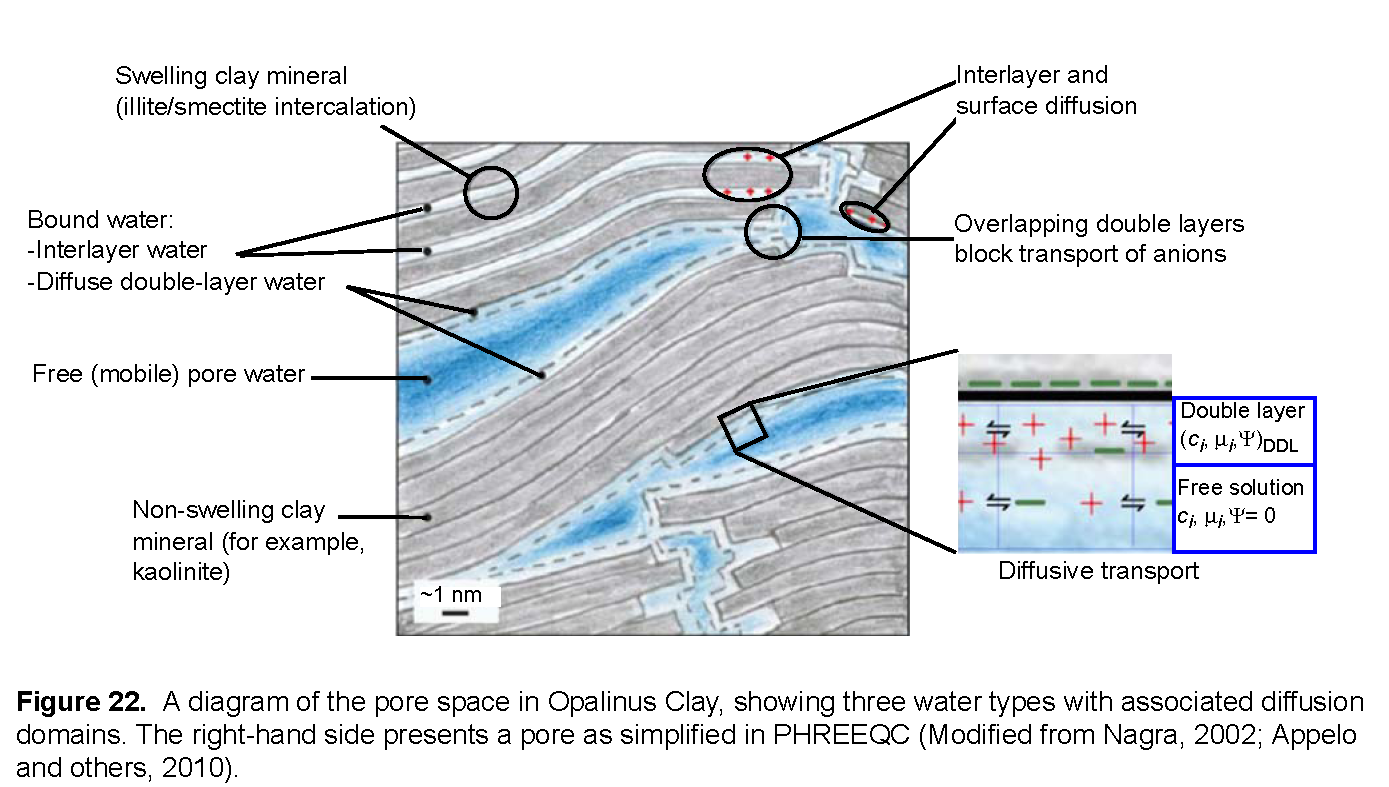For calculating diffusion, one needs to account for the different diffusion coefficients of the tracers, the effect of the filters, and the properties of the clay. Figure 22 presents a schematic diagram of the processes in the clay (Appelo and others, 2010). The pores in the clay are lined with clay minerals that have a negative surface charge. The charge is partly neutralized by cations that are bound to the surface and partly by the electrostatic double layer that extends some distance into the pore. The double layer contains an excess of cations (counter-ions, in general) and a deficit of anions (co-ions, in general). In swelling clay minerals, such as montmorillonite, cations in the interlayer space also neutralize part of the negative charge. In comparison with concentration gradients that drive diffusion in free (uncharged) pore water, the gradient for a cation is magnified in the double layer, whereas the gradient for an anion is diminished, and the gradient for a neutral species remains the same. The charge in the double layer lowers the dielectric permittivity of the water, which enhances the ion-association of cations and anions into neutral species. Also, the viscosity of water may be higher than in free pore water. Where double layers overlap in pore constrictions, anions are impeded and forced to take longer paths, whereas cations and neutral species pass through unhindered.

PHREEQC has capabilities to model many of these diffusion processes. An averaged double layer composition can be calculated by using the identifier -Donnan in keyword SURFACE, which, in essence, neutralizes the surface charge. Solute species can be assigned an enrichment factor in the Donnan pore space to emulate the additional concentration change as a result of different ion association. For diffusion, the viscosity can be set differently with respect to free pore water. In this example, all these properties are adjusted for the tracers tritium (HTO), Na+, Cs+ and Cl- to simulate experimental results.

The experiments to be modeled were done in a radial diffusion cell, shown in figure 23. The radial cell enables measurement of diffusion parallel to the bedding plane of the clay (Van Loon and others, 2004). A solution with tracers is circulated at the surface of the inner filter, and another solution with the same major ions, but without tracers, contacts the surface of the outer filter. The latter solution is removed regularly and analyzed for the tracer that has diffused through the filters and the clay. Meanwhile, the outer solution is replaced by fresh solution, thus, maintaining tracer concentrations near zero in the outer solution. In the simulations, low solubility phases were defined to maintain small concentrations of tracers in the outer solution. The moles of precipitates of these phases give the accumulated mass that has diffused through the column.

For a linear column, PHREEQC calculates diffusion automatically by using the parameters entered with identifier -multi_D in keyword TRANSPORT. However, diffusion in the experiment is radial, and the filters have different properties than the clay. Also, the boundary solutions for the default (linear) column have a constant composition, whereas we want to know the concentration changes in these solutions during the diffusion experiment. All these experimental details can be simulated for a stagnant column by defining mixing factors among the individual cells with keyword MIX.

The mixing factors can be derived from Fick’s diffusion equations,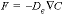and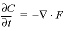, which transform to finite differences for an arbitrarily shaped cell j: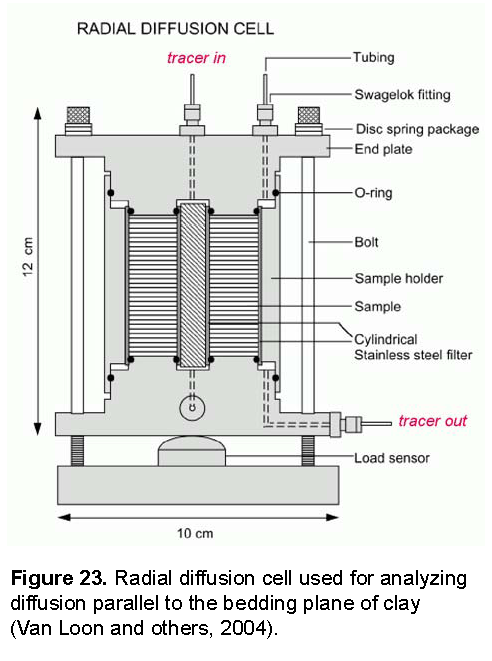###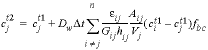, (44)

whereis the concentration in cell j at the current time (mol/m3),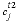is the concentration in cell j after the time step, Dw is the tracer diffusion coefficient (m2/s),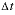is the time step (s), i is an adjacent cell,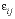is the porosity over the interface of cells i and j (unitless), Gij is the geometrical factor that corrects for tortuosity of the porous medium (unitless), hij is the distance between midpoints of the cells (m), Aij is the shared surface area of cells i and j (m2), Vj is the volume of cell j (m3), and fbc is a factor for boundary cells (unitless). The summation is for all cells (up to n) adjacent to j. When hij is equal for all cells, a central difference algorithm is obtained that has second-order accuracy [O(h)2] (Appelo and others, 2008). It is therefore advantageous to make the grid regular. However, the same accuracy is achievable for a heterogeneous domain, even with widely variable gridsize, if the harmonic mean of the parameters in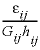is used. These parameters together, translate the tracer diffusion coefficient Dw into the effective diffusion coefficient De.

The harmonic mean can be derived in general (omitting the activity coefficient to simplify the formulas) as follows. The fluxes inside cells i and j and over the interface of the two cells must be the same and are given by:

###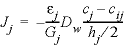, (47)

where h is the cell length (m), and cij is the concentration at the interface. Substituting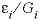= gi, in equation 46 and similarly in equation 47, the two equations can be combined while eliminating the concentration cij to give:

###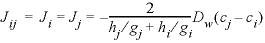. (48)

Multiplying the flux by the surface area, the time step, and the boundary factor, and dividing by the volume of the cell for which the concentration change is calculated (here, cell j), the mixing factor is obtained:

###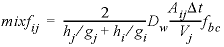, (49)

where fbc is 2 for cells in contact with a constant concentration cell, and 1 otherwise. When calculating mixf, Vj is set to 10 -3 m 3 , and for Dw the default diffusion coefficient is used, entered with identifier -multi_D. In multicomponent-diffusion mode, PHREEQC adapts the volume Vj (entered as 10 -3 m 3 in equation 49), to the actual volume of water in cell j, and multiplies the mixing factor for each solute species a by the ratio of the tracer diffusion coefficient for a and the default diffusion coefficient (Dw, a / Dw).

To avoid numerical oscillations, it is necessary that mixf < 0.5, which can be realized by limiting the time step. This maximum permissible time step is usually determined by the cell with the smallest volume (in the model) and the fluxes of the proton, which normally has the highest tracer diffusion coefficient. However, if the proton concentration is sufficiently buffered by alkalinity or other species, and the solutions are uniform except for the tracers, the tracer with the highest Dw may be selected for calculating the maximum permissible time step. If, nevertheless, time steps are too large, PHREEQC warns that negative concentrations are calculated (and the program stops because the system reached an infeasible state), the time step can be subdivided. For example, -time_step 5e2 3 will subdivide the time step of 500 s in three equal ones of 166.7 s.

The input file in table 60 defines the physical and chemical properties of the clay pore space and writes the mixing factors for diffusional transport in the filters and the clay. The filters used by Van Loon have a geometrical factor of 4 for all the tracers, whether charged or not (Glaus and others, 2008). In the clay, the geometrical factor for HTO is 6.2. For cations, the geometrical factor appears to be 2 to 4 times smaller than for tritium. However, in this example (example 21), the same geometrical factor is used for both HTO and the cations; the smaller apparent geometrical factors are modeled by subdividing the pore space into free pore water and double-layer water. The concentrations of cations are higher in double-layer water than in free pore water, and hence, the concentration gradients of cations are also higher, which, as noted previously enhances their diffusion. In models that do not account for this physical aspect of the clay pore space, the faster diffusion is treated by diminishing the geometrical factor.

For anions, the geometrical factor is about 1.5 times larger than for tritium. This larger factor is related to narrowing of the pores, where overlapping double layers decrease the accessible porosity for anions and obstruct their passage (as noted above). The model accounts for the observed, smaller accessible porosity for anions relative to tritium and cations by the calculation of anion exclusion in the double layer.

The file starts with the tracer species, where, for the monovalent tracers 22Na+ (=“Na_tr+”) and Cs+, an enrichment factor for the double layer is entered with -erm_ddl in the SOLUTION_SPECIES data block. The enrichment is related to increased complexation of the polyvalent cations in the low dielectric permittivity of the double layer, and thus, more charge must be counterbalanced by monovalent cations. Sorption of Cs+ is much stronger than that of Na+, which is modeled by two surface complexes and one exchange reaction with large constants. The constants are based on the measured adsorption isotherm for Opalinus Clay, but may be generally applicable because they are associated primarily with strong sorption sites on illite. Next, the file writes SOLUTION 0-2 for a regular column, followed by SOLUTION 3,

 `TITLE Diffusion through Opalinus Clay in a radial diffusion cell, ` ` Appelo and others, 2010, GCA, v. 74, p. 1201-1219.` `SOLUTION_MASTER_SPECIES` `# element species alk gfw_formula element_gfw` ` Hto Hto 0.0 20 20` ` Na_tr Na_tr+ 0.0 22 22` ` Cl_tr Cl_tr- 0.0 36 36` ` Cs Cs+ 0.0 132.905 132.905` `SOLUTION_SPECIES` ` Hto = Hto; log_k 0; -gamma 1e6 0; -dw 2.236e-9` ` Na_tr+ = Na_tr+; log_k 0; -gamma 4.0 0.075; -dw 1.33e-9; -erm_ddl 1.23` ` Cl_tr- = Cl_tr-; log_k 0; -gamma 3.5 0.015; -dw 1.31e-9 # dw = dw(water) / 1.55 = 2.03e-9 / 1.55` ` Cs+ = Cs+; log_k 0; -gamma 3.5 0.015; -dw 2.07e-9; -erm_ddl 1.23` `SURFACE_MASTER_SPECIES` ` Su_fes Su_fes- # Frayed Edge Sites` ` Su_ii Su_ii- # Type II sites of intermediate strength` ` Su_ Su_- # Double layer, planar sites are modeled with EXCHANGE` `SURFACE_SPECIES` ` Su_fes- = Su_fes-; log_k 0` ` Na+ + Su_fes- = NaSu_fes; log_k 10` ` Na_tr+ + Su_fes- = Na_trSu_fes; log_k 10` ` K+ + Su_fes- = KSu_fes; log_k 12.4` ` Cs+ + Su_fes- = CsSu_fes; log_k 17.14` ` ` ` Su_ii- = Su_ii-; log_k 0` ` Na+ + Su_ii- = NaSu_ii; log_k 10` ` Na_tr+ + Su_ii- = Na_trSu_ii; log_k 10` ` K+ + Su_ii- = KSu_ii; log_k 12.1` ` Cs+ + Su_ii- = CsSu_ii; log_k 14.6` ` ` ` Su_- = Su_-; log_k 0` ` ` `EXCHANGE_SPECIES` ` Na_tr+ + X- = Na_trX; log_k 0.0; -gamma 4.0 0.075` ` Cs+ + X- = CsX; log_k 2.04; -gamma 3.5 0.015` ` ` `SOLUTION 0-2 column with only cell 1, two boundary solutions 0 and 2.` ` Na 1; Cl 1` `END` ` ` `KNOBS; -diagonal_scale true # -tolerance 1e-20 # because of low concentrations` ` ` `SOLUTION 3 tracer solution` ` pH 7.6; pe 14 O2(g) -1.0; temp 23` ` Na 240; K 1.61; Mg 16.9; Ca 25.8; Sr 0.505` ` Cl 300; S(6) 14.1; Fe(2) 0.0; Alkalinity 0.476` `# uncomment tracer concentrations and kg water 1 by 1...` ` Hto 1.14e-6; -water 0.2` `# Cl_tr 2.505e-2; -water 0.502` `# Cs 1; Na_tr 1.87e-7; -water 1.02` `SELECTED_OUTPUT` ` -file radial; -reset false` `USER_PUNCH` ` # Define symbols and pi...` `1 nl\$ = EOL\$ # newline` `2 x\$ = CHR\$(35) # cross '#'` `3 sc\$ = CHR\$(59) # semicolon ';'` `4 pi = 2 * ARCTAN(1e10) # 3.14159...` ` ` ` # Define experimental parameters...` `10 height = 0.052 # length of the clay cylinder / m` `20 r_int = 6.58e-3 # inner radius of clay cylinder / m` `30 r_ext = 25.4e-3 # outer radius` `40 thickn_filter1 = 1.8e-3 # tracer-in filter thickness / m` `50 thickn_filter2 = 1.6e-3 # tracer-out filter thickness / m` `60 por_filter1 = 0.418 # porosity` `70 por_filter2 = 0.367` `80 G_filter1 = 4.18 # geometrical factor. (for filters, G = por / 10)` `90 G_filter2 = 3.67` `100 V_end = 0.2 # volume of the tracer-out solution / L` `110 thickn_clay = r_ext - r_int # clay thickness / m` `120 por_clay = 0.159` `130 rho_b_eps = 2.7 * (1 - por_clay) / por_clay # clay bulk density / porosity / (kg/L)` `140 CEC = 0.12 * rho_b_eps # CEC / (eq/L porewater)` `150 A_por = 37e3 * rho_b_eps # pore surface area / (m2/L porewater)` ` ` `160 DIM tracer\$(4), exp_time(4), scale_y1\$(4), scale_y2\$(4), profile_y1\$(4), profile_y2\$(4)` `170 DATA 'Hto', 'Cl_tr', 'Na_tr', 'Cs'` `180 READ tracer\$(1), tracer\$(2), tracer\$(3), tracer\$(4)` ` # experimental times (seconds) for HTO, 36Cl, 22Na and Cs, respectively,` ` # in order of increasing times...` `200 DATA 86400 * 20, 86400 * 40, 86400 * 45, 86400 * 1000` `210 READ exp_time(1), exp_time(2), exp_time(3), exp_time(4)` ` # scale y1-axis (flux) (not used)...` `230 DATA '1', '1', '1', '1'` `240 READ scale_y1\$(1), scale_y1\$(2), scale_y1\$(3), scale_y1\$(4)` ` # scale y2-axis (mass) (not used)...` `260 DATA '1', '1', '1', '1'` `270 READ scale_y2\$(1), scale_y2\$(2), scale_y2\$(3), scale_y2\$(4)` ` # scale max of the profile y axes...` `280 DATA '0 1.2e-9', '0 2.5e-5', '0 2e-10', '0 auto'` `290 READ profile_y1\$(1), profile_y1\$(2), profile_y1\$(3), profile_y1\$(4)` `300 DATA '0 1.2e-9', '0 2.5e-5', '0 6e-10', '0 auto'` `310 READ profile_y2\$(1), profile_y2\$(2), profile_y2\$(3), profile_y2\$(4)` ` ` ` # Define model parameters...` `350 Dw = 2.5e-9 # default tracer diffusion coefficient / (m2/s)` `360 nfilt1 = 1 # number of cells in filter 1` `370 nfilt2 = 1 # number of cells in filter 2` `380 nclay = 11 # number of clay cells` `390 f_free = 0.117 # fraction of free pore water (0.01 - 1)` `400 f_DL_charge = 0.45 # fraction of CEC charge in electrical double layer` `410 tort_n = -0.99 # exponent in Archie's law, -1.045 without filters` `420 G_clay = por_clay^tort_n # geometrical factor` `430 interlayer_D\$ = 'false' # 'true' or 'false' for interlayer diffusion` `440 G_IL = 700 # geometrical factor for clay interlayers` `450 punch_time = 60 * 60 * 6 # punch time / seconds` `460 profile\$ = 'true' # 'true' or 'false' for c/x profile visualization` `470 IF nfilt1 = 0 THEN thickn_filter1 = 0` `480 IF nfilt2 = 0 THEN thickn_filter2 = 0` ` ` ` # See which tracer is present...` `490 IF tot("Hto") > 1e-10 THEN tracer = 1 ELSE \` ` IF tot("Cl_tr") > 1e-10 THEN tracer = 2 ELSE tracer = 3` ` ` ` # Define clay pore water composition...` `520 sol\$ = nl\$ + ' pH 7.6' + sc\$ +' pe 14 O2(g) -1.0' + sc\$ +' temp 23'` `530 sol\$ = sol\$ + nl\$ + ' Na 240' + sc\$ +' K 1.61' + sc\$ +' Mg 16.9' + sc\$ +' Ca 25.8' + sc\$ +' Sr 0.505'` `540 sol\$ = sol\$ + nl\$ + ' Cl 300' + sc\$ +' S(6) 14.1' + sc\$ +' Fe(2) 0.0' + sc\$ +' Alkalinity 0.476'` ` ` ` # Define phases in which the tracers precipitate...` `550 tracer_phases\$ = nl\$ + 'PHASES '` `560 tracer_phases\$ = tracer_phases\$ + nl\$ + ' A_Hto' + nl\$ + ' Hto = Hto' + sc\$ +' log_k -15'` `570 tracer_phases\$ = tracer_phases\$ + nl\$ + ' A_Na_tr' + nl\$ + ' Na_trCl = Na_tr+ + Cl-' + sc\$ + ' log_k -14'` `580 tracer_phases\$ = tracer_phases\$ + nl\$ + ' A_Cl_tr' + nl\$ + ' NaCl_tr = Na+ + Cl_tr-' + sc\$ +' log_k -14'` `590 tracer_phases\$ = tracer_phases\$ + nl\$ + ' A_Cs' + nl\$ + ' CsCl = Cs+ + Cl-' + sc\$ + ' log_k -13'` `600 DIM tracer_equi\$(4)` `610 FOR i = 1 TO 4` `620 tracer_equi\$(i) = nl\$ + 'A_' + tracer\$(i) + ' 0 0'` `630 NEXT i` ` ` ` # Write solutions for the cells...` `650 punch nl\$ + 'PRINT ' + sc\$ + ' -reset false' + sc\$ + ' -echo_input true' + sc\$ + ' -user_print true'` `660 IF nfilt1 = 0 THEN GOTO 800` `670 punch nl\$ + x\$ + ' filter cells at tracer-in side...'` `680 r1 = r_int - thickn_filter1` `690 xf1 = thickn_filter1 / nfilt1` `700 FOR i = 1 TO nfilt1` `710 num\$ = TRIM(STR\$(i + 3)) + sc\$` `720 V_water = 1e3 * height * por_filter1 * pi * (SQR(r1 + xf1) - SQR(r1))` `730 punch nl\$ + 'SOLUTION ' + num\$ + ' -water ' + STR\$(V_water)` `740 punch sol\$ + nl\$` `750 r1 = r1 + xf1` `760 NEXT i` ` ` `800 punch nl\$ + nl\$ + x\$ + ' cells in Opalinus Clay...'` `810 r1 = r_int` `820 x = thickn_clay / nclay` `830 FOR i = 1 TO nclay` `840 num\$ = TRIM(STR\$(i + 3 + nfilt1)) + sc\$` `850 V_water = 1e3 * height * por_clay * pi * (SQR(r1 + x) - SQR(r1))` `860 punch nl\$ + 'SOLUTION ' + num\$ + ' -water ' + STR\$(V_water * f_free)` `870 punch sol\$` `880 IF f_free = 1 and tracer = 1 THEN GOTO 960` `890 punch nl\$ + 'SURFACE ' + num\$ + ' -equil ' + num\$` `900 punch nl\$ + ' Su_ ' + TRIM(STR\$(f_DL_charge * CEC * V_water)) + STR\$(A_por) + ' ' + STR\$(V_water)` `910 punch nl\$ + ' Su_ii ' + TRIM(STR\$(7.88e-4 * rho_b_eps * V_water))` `920 punch nl\$ + ' Su_fes ' + TRIM(STR\$(7.4e-5 * rho_b_eps * V_water))` `930 IF f_free < 1 THEN punch nl\$ + ' -Donnan ' + TRIM(STR\$((1 - f_free) * 1e-3 / A_por))` `940 punch nl\$ + 'EXCHANGE ' + num\$ + ' -equil ' + num\$` `950 punch nl\$ + ' X ' + TRIM(STR\$((1 - f_DL_charge) * CEC * V_water)) + nl\$` `960 r1 = r1 + x` `970 NEXT i` ` ` `1000 IF nfilt2 = 0 THEN GOTO 1200` `1010 punch nl\$ + nl\$ + x\$ + ' tracer-out filter cells...'` `1020 r1 = r_ext` `1030 xf2 = thickn_filter2 / nfilt2` `1040 FOR i = 1 TO nfilt2` `1050 num\$ = TRIM(STR\$(i + 3 + nfilt1 + nclay)) + sc\$` `1060 V_water = 1e3 * height * por_filter2 * pi * (SQR(r1 + xf2) - SQR(r1))` `1070 punch nl\$ + 'SOLUTION ' + num\$ + ' -water ' + STR\$(V_water)` `1080 punch sol\$ + nl\$` `1090 r1 = r1 + xf2` `1100 NEXT i` ` ` `1200 punch nl\$ + x\$ + ' outside solution...'` `1210 num\$ = TRIM(STR\$(4 + nfilt1 + nclay + nfilt2)) + sc\$` `1220 punch nl\$ + 'SOLUTION ' + num\$ + ' -water ' + STR\$(V_end)` `1230 punch sol\$` `1240 punch nl\$ + 'END'` ` ` ` # Write phases in which the tracers precipitate...` `1300 punch nl\$ + tracer_phases\$` `1310 punch nl\$ + 'EQUILIBRIUM_PHASES ' + num\$ + tracer_equi\$(tracer)` `1312 If tracer = 3 THEN punch nl\$ + tracer_equi\$(tracer + 1)` `1320 punch nl\$ + 'END'` ` ` ` # Define mixing factors for the diffusive flux between cells 1 and 2:` ` # J_12 = -2 * Dw / (x_1 / g_1 + x_2 / g_2) * (c_2 - c_1)` ` # Multiply with dt * A / (V = 1e-3 m3). (Actual volumes are given with SOLUTION; -water)` ` # Use harmonic mean: g_1 = por_1 / G_1, g_2 = por_2 / G_2, x_1 = Delta(x_1), etc.` `1400 IF nfilt1 > 0 THEN gf1 = por_filter1 / G_filter1` `1410 IF nfilt2 > 0 THEN gf2 = por_filter2 / G_filter2` `1420 g = por_clay / G_clay` ` # Find max time step = 0.5 * V_water * dx * G_factor / (Dw * por * A * fbc)` ` # V_water = por * pi * height * ((r + dr)^2 - r^2)` ` # A = por * pi * height * r * 2` ` # At the inlet of the tracers, fbc = 2...` `1500 IF nfilt1 = 0 THEN GOTO 1530` `1510 r1 = r_int - thickn_filter1` `1520 ff = (SQR(r1 + xf1) - SQR(r1)) * xf1 * G_filter1 / (r1 * 2) / 2` `1530 ff1 = (SQR(r_int + x) - SQR(r_int)) * x * G_clay / (r_int * 2) / 2` ` # Perhaps the clay has very small cells...` `1540 IF nfilt1 = 0 THEN ff = ff1 ELSE IF ff1 * 2 < ff THEN ff = ff1 * 2` ` # Or at the filter1-clay transition, fbc = 1...` `1550 IF nfilt1 > 0 THEN ff1 = (SQR(r_int + x) - SQR(r_int)) * (xf1 / gf1 + x / g) / (2 * r_int * 2)` `1560 IF nfilt1 > 0 AND ff1 < ff THEN ff = ff1` ` # Perhaps filter2 has very small cells...` `1570 IF nfilt2 > 0 THEN ff1 = (SQR(r_ext + xf2) - SQR(r_ext)) * xf2 * G_filter2 / (r_ext * 2)` `1580 IF nfilt2 > 0 AND ff1 < ff THEN ff = ff1` `1590 dt_max = 0.5 * ff / Dw` ` # Check with punch times, set shifts...` `1610 IF punch_time < dt_max THEN dt = punch_time ELSE dt = dt_max` `1620 punch_fr = 1` `1630 IF dt < punch_time THEN punch_fr = ceil(punch_time / dt)` `1640 dt = punch_time / punch_fr` `1650 shifts = ceil(exp_time(tracer) / dt)` ` # Write mixing factors...` `1700 punch nl\$ + nl\$ + x\$ + ' mixing factors...'` `1710 r1 = r_int` `1720 IF nfilt1 > 0 THEN r1 = r_int - thickn_filter1` `1730 A = height * 2 * pi` `1740 FOR i = 0 TO nfilt1 + nclay + nfilt2` `1750 IF i = 0 OR i = nfilt1 + nclay + nfilt2 THEN fbc = 2 ELSE fbc = 1` `1760 IF i > nfilt1 OR nfilt1 = 0 THEN GOTO 1810` `1770 IF i < nfilt1 THEN mixf = Dw * fbc / (xf1 / gf1) * dt * A * r1 / 1e-3` `1780 IF i = nfilt1 THEN mixf = 2 * Dw / (xf1 / gf1 + x / g) * dt * A * r1 / 1e-3` `1790 IF i < nfilt1 THEN r1 = r1 + xf1 ELSE r1 = r1 + x` `1800 GOTO 1880` `1810 IF i > nfilt1 + nclay THEN GOTO 1860` `1820 mixf = Dw * fbc / (x / g) * dt * A * r1 / 1e-3` `1830 IF i = nfilt1 + nclay AND nfilt2 > 0 THEN mixf = 2 * Dw / (xf2 / gf2 + x / g) * dt * A * r1 / 1e-3` `1840 IF i < nfilt1 + nclay THEN r1 = r1 + x ELSE r1 = r1 + xf2` `1850 GOTO 1880` `1860 mixf = Dw * fbc / (xf2 / gf2) * dt * A * r1 / 1e-3` `1870 r1 = r1 + xf2` `1880 punch nl\$ + 'MIX ' + TRIM(STR\$(i + 3)) + sc\$ + STR\$(i + 4) + STR\$(mixf)` `1890 NEXT i` `1900 punch nl\$ + 'END'` ` ` ` # Write TRANSPORT...` `2000 punch nl\$ + 'TRANSPORT'` `2010 stag = 2 + nfilt1 + nclay + nfilt2` `2020 punch nl\$ + ' -warnings true'` `2030 punch nl\$ + ' -shifts ' + TRIM(STR\$(shifts))` `2040 punch nl\$ + ' -flow diff' + sc\$ + ' -cells 1' + sc\$ + ' -bcon 1 2' + sc\$ + ' -stag ' + TRIM(STR\$(stag))` `2050 punch nl\$ + ' -time ' + STR\$(dt)` `2060 punch nl\$ + ' -multi_D true ' + STR\$(Dw) + STR\$(por_clay) + ' 0.0 ' + TRIM(STR\$(-tort_n))` `2070 punch nl\$ + ' -interlayer_D ' + interlayer_D\$ + ' 0.001 0.0 ' + TRIM(STR\$(G_IL))` `2080 punch nl\$ + ' -punch_fr ' + TRIM(STR\$(punch_fr)) + sc\$ + ' -punch_c ' + TRIM(STR\$(2 + stag))` ` ` ` # Write USER_GRAPH...` `2180 FOR i = 0 to 1` `2190 punch nl\$ + 'USER_GRAPH ' + TRIM(STR\$(tracer + i)) + ' Example 21' + nl\$` `2200 punch nl\$ + ' -chart_title " ' + tracer\$(tracer + i) + ' Diffusion to Outer Cell"'` `2210 punch nl\$ + ' -plot_tsv_file ex21_' + tracer\$(tracer + i) + '_rad.tsv'` `2220 punch nl\$ + ' -axis_scale x_axis 0 ' + TRIM(STR\$(exp_time(tracer + i) / (3600 * 24)))` `2230 punch nl\$ + ' -axis_titles "Time, in days" "Flux, in moles per square meter per second" \` ` "Accumulated mass, in moles"'` `2240 punch nl\$ + ' -plot_concentration_vs time'` `2250 punch nl\$ + ' 10 days = total_time / (3600 * 24)'` `2260 punch nl\$ + ' 20 a = equi("A_' + tracer\$(tracer + i) + '")'` `2270 punch nl\$ + ' 30 IF get(1) = 0 AND total_time > 0 THEN put(total_time, 1)'` `2280 punch nl\$ + ' 40 dt = get(1)'` `2290 A = 2 * pi * r_ext * height` `2300 i\$ = TRIM(STR\$(2 + i))` `2310 punch nl\$ + ' 50 plot_xy days - dt / (2 * 3600 * 24), (a - get(' + i\$ + ')) / dt /' + STR\$(A) + \` ` ', color = Green, symbol = None'` `2320 punch nl\$ + ' 60 put(a, ' + i\$ + ')'` `2330 punch nl\$ + ' 70 plot_xy days, equi("A_' + tracer\$(tracer + i) + \` ` '"), y_axis = 2, color = Red, symbol = None'` `2340 IF tracer < 3 THEN GOTO 2360` `2350 NEXT i` `2360 punch nl\$ + 'END'` ` ` `2400 IF profile\$ = 'true' THEN GOSUB 3000` `2410 IF tracer < 3 THEN END # finished for Hto and Cl` ` ` ` # Continue with Cs...` `2420 IF profile\$ = 'false' THEN punch nl\$ + 'USER_GRAPH ' + TRIM(STR\$(tracer)) + sc\$ + ' -detach' ELSE \` ` punch nl\$ + 'USER_GRAPH ' + TRIM(STR\$(tracer + 4)) + sc\$ + ' -detach'` `2440 tracer = tracer + 1` `2450 punch nl\$ + 'TRANSPORT'` `2460 shifts = ceil((exp_time(tracer) - exp_time(tracer - 1))/ dt)` `2480 punch nl\$ + ' -shifts ' + TRIM(STR\$(shifts))` `2490 punch nl\$ + ' -punch_fr ' + TRIM(STR\$(punch_fr)) + sc\$ + ' -punch_c ' + TRIM(STR\$(2 + stag))` `2500 punch nl\$ + 'END'` `2510 IF profile\$ = 'true' THEN GOSUB 3000` `2520 END # finished...` ` ` ` # Write TRANSPORT and USER_GRAPH for concentration profile...` `3000 punch nl\$ + 'TRANSPORT'` `3010 punch nl\$ + ' -shifts 0'` `3020 punch nl\$ + ' -punch_fr 2' + sc\$ + ' -punch_c 3-' + TRIM(STR\$(2 + stag))` ` # Write USER_GRAPH...` `3030 punch nl\$ + 'USER_GRAPH ' + TRIM(STR\$(tracer)) + sc\$ + ' -detach'` `3040 punch nl\$ + 'USER_GRAPH ' + TRIM(STR\$(tracer + 4)) + ' Example 21' + nl\$` `3050 punch nl\$ + ' -chart_title "' + tracer\$(tracer) + ' Concentration Profile: Filter1 | Clay | Filter2"'` `3060 REM punch nl\$ + ' -plot_tsv_file + tracer\$(tracer) + '_prof.tsv'` `3070 punch nl\$ + ' -axis_scale x_axis 0 ' + TRIM(STR\$((thickn_filter1 + thickn_clay + thickn_filter2) * 1e3))` `3080 punch nl\$ + ' -axis_scale y_axis ' + profile_y1\$(tracer)` `3090 punch nl\$ + ' -axis_scale sy_axis ' + profile_y2\$(tracer)` `3100 punch nl\$ + ' -axis_titles ' + '"Distance, in millimeters" "Free pore-water molality" "Total molality"'` `3110 punch nl\$ + ' -headings ' + tracer\$(tracer) + '_free ' + tracer\$(tracer) + '_tot'` `3120 punch nl\$ + ' -plot_concentration_vs x'` `3130 punch nl\$ + ' -initial_solutions true'` `3140 punch nl\$ + ' 10 IF cell_no = 3 THEN xval = 0 ELSE xval = get(14)'` `3150 punch nl\$ + ' 20 IF (' + TRIM(STR\$(nfilt1)) + ' = 0 OR cell_no > ' + TRIM(STR\$(nfilt1 + 3)) + ') THEN GOTO 60'` `3160 punch nl\$ + ' 30 IF (cell_no = 4) THEN xval = xval + 0.5 * ' + TRIM(STR\$(xf1))` `3170 punch nl\$ + ' 40 IF (cell_no > 4 AND cell_no < ' + TRIM(STR\$(nfilt1 + 4)) + \` ` ') THEN xval = xval + ' + TRIM(STR\$(xf1))` `3180 punch nl\$ + ' 50 GOTO 200'` `3190 punch nl\$ + ' 60 IF (cell_no = ' + TRIM(STR\$(4 + nfilt1)) + ') THEN xval = xval + 0.5 * ' + \` ` TRIM(STR\$(xf1)) + ' + 0.5 * ' + TRIM(STR\$(x))` `3200 punch nl\$ + ' 70 IF (cell_no > ' + TRIM(STR\$(4 + nfilt1)) + ' AND cell_no < ' + \` ` TRIM(STR\$(4 + nfilt1 + nclay)) + ') THEN xval = xval + ' + TRIM(STR\$(x)) + ' ELSE GOTO 90'` `3210 punch nl\$ + ' 80 GOTO 200'` `3220 punch nl\$ + ' 90 IF (cell_no = ' + TRIM(STR\$(4 + nfilt1 + nclay)) + ') THEN xval = xval + 0.5 * ' + \` ` TRIM(STR\$(x)) + ' + 0.5 * ' + TRIM(STR\$(xf2))` `3230 punch nl\$ + ' 100 IF (cell_no > ' + TRIM(STR\$(4 + nfilt1 + nclay)) + ' AND cell_no <= ' + \` ` TRIM(STR\$(3 + nfilt1 + nclay + nfilt2)) + ') THEN xval = xval + ' + TRIM(STR\$(xf2))` `3240 punch nl\$ + ' 110 IF (cell_no = ' + TRIM(STR\$(4 + nfilt1 + nclay + nfilt2)) + \` ` ') THEN xval = xval + 0.5 * ' + TRIM(STR\$(xf2))` `3250 punch nl\$ + ' 200 y1 = TOT("' + tracer\$(tracer) + '")'` `3260 punch nl\$ + ' 210 plot_xy xval * 1e3, y1, color = Blue, symbol = Plus'` `3270 punch nl\$ + ' 220 IF cell_no = 3 THEN put(y1, 15)'` `3280 punch nl\$ + ' 230 IF (cell_no < ' + TRIM(STR\$(4 + nfilt1)) + ' OR cell_no > ' + \` ` TRIM(STR\$(3 + nfilt1 + nclay)) + ') THEN GOTO 400'` `3290 punch nl\$ + ' 240 y2 = SYS("' + tracer\$(tracer) + '") / (tot("water") + edl("water"))'` ` # Remove REM if total conc's per kg solid must be plotted (and adapt axis_titles)...` `3310 punch nl\$ + ' 250 REM y2 = y2 / ' + TRIM(STR\$(rho_b_eps)) + x\$ + ' conc / kg solid'` `3320 punch nl\$ + ' 260 plot_xy xval * 1e3, y2, symbol = Circle, y_axis = 2'` `3330 punch nl\$ + ' 270 IF (cell_no > ' + TRIM(STR\$(5 + nfilt1)) + ') THEN GOTO 400'` `3340 punch nl\$ + ' 280 IF ' + TRIM(STR\$(nfilt1)) + ' THEN plot_xy ' + TRIM(STR\$(thickn_filter1 * 1e3)) + \` ` ', get(15), color = Black, symbol = None'` `3350 punch nl\$ + ' 290 IF ' + TRIM(STR\$(nfilt2)) + ' THEN plot_xy ' + \` ` TRIM(STR\$((thickn_filter1 + thickn_clay) * 1e3)) + ', get(15), color = Black, symbol = None'` `3360 punch nl\$ + ' 300 put(0, 15)'` `3370 punch nl\$ + ' 400 put(xval, 14)'` `3380 punch nl\$ + 'END'` `3390 RETURN` `END` `PRINT` ` -selected_output false; -status false` `INCLUDE\$ radial` `END`

which forms the start of the stagnant column and circulates at the inner filter of the diffusion cell. This solution contains the tracers and the amounts of water used in the experiment. The lines can be uncommented one by one to run the file successively with the different tracers and amounts of water. When SOLUTION 3 is calculated, USER_PUNCH is processed to write a SELECTED_OUTPUT file named radial, which contains the SOLUTION definitions for the cells in the filters and the clay, the mixing factors, and the TRANSPORT and USER_GRAPH data blocks. The Basic lines in USER_PUNCH do the following tasks:

• Lines 1-4 define variables that facilitate printing of special symbols used in printing the output--end of line, comment sign, and semicolon--and define π, which is used to write definitions for the radial configuration of the experimental cell.
• Lines 10-150 define the dimensions of the experimental cell and properties of the filters and the clay that have been measured and, thus, should be considered as constant.
• Lines 160-310 fill arrays with the names of the tracers, the experimental times, and the scale factors for the graphs.
• Lines 350-480 give model parameters that may be varied to simulate the experiments, and can be changed to check the diffusion model. Typically, for checking the numerics, the number of cells for the filters (variables nfilt1 and nfilt2) and for the clay (nclay), and the time step (punch_time) can be altered without affecting the calculated results. It also is interesting to set nfilt1 and/or nfilt2 to zero and inspect the effects that the filters have on the fluxes. (The program will fail if nclay is set to zero.) Values of the other model parameters were derived from the geometrical factors obtained by fitting the measured tracer diffusion curves (Appelo and others, 2010). By adjusting these parameters, the Basic program and the functioning of PHREEQC can be verified. For example, f_free sets the fraction of free pore water, partitioning the pore space in free and double layer water. Values may range from 0.01 to 1. The variable has little effect on tritium which, as an uncharged species, diffuses equally quickly in free and double-layer water (if the latter is given the viscosity of free pore water). However, the variable has a major effect on the diffusion of 36Cl-, which diffuses more quickly in the free pore water. The parameter f_DL_charge partitions the Cation Exchange Capacity (CEC) over the double layer and exchange sites. Increasing its value does not affect the diffusion of tritium, but decreases the flux of Cl- and increases the flux of Na+.
• Line 490 checks which tracer is present to set the experimental times and scale factors for the graphs.
• Lines 520-540 define clay pore-water composition.
• Lines 550-630 define the solid phases in which the tracers precipitate in the outer solution. The moles of this phase will record the amounts that have diffused. The phases have such a low solubility that the tracer concentration is essentially zero.
• Lines 650-1100 write the solutions for the filter cells and the clay, with radially increasing amounts of water. For the clay, keyword SURFACE is used to define the moles of the surface sites of Su_, Su_ii and Su_fes, for the double layer, and the sites on illite that sorb the alkaline cations with intermediate and high strength, respectively. The fixed sites of the Cation Exchange Capacity (CEC) are defined with EXCHANGE.
• Lines 1200-1320 write the external SOLUTION and the EQUILIBRIUM_PHASES in which the tracers are captured.
• Lines 1400-1900 calculate and write the mixing factors as explained above in equations 44-49. First, the maximum time step is derived from one of the following: the innermost filter cell, at the transition of the inner filter to the clay, the innermost clay cell, or the outer filter cell. The time step is decreased when it is larger than desired by punch_time. With this time step, the mixing factors are calculated for each cell and written to the file, taking care of the heterogeneities at physical boundaries and the radial outline of the field.
• Lines 2000-2520 write data blocks for TRANSPORT and USER_GRAPH. The experimental data (L.R. Van Loon, Paul Scherrer Institute, written commun., 2011) are plotted (“-plot_tsv_file file_name”) together with the calculated accumulated mass in the outer solution and the flux, obtained by dividing the mass that has accumulated by the time interval and the outer surface area of the clay.
• Lines 3000-3390 contain the subroutine to write TRANSPORT and USER_GRAPH data blocks for plotting concentration profiles in the filters and the clay.

The experiments with the tracers 22Na and Cs can be calculated together, because the tracer solutions contained identical amounts of water. Thus, when the 22Na profile has been plotted after 45 days, another TRANSPORT block is written to calculate diffusion of Cs during 1,000 days in total.

Following the END after USER_PUNCH, the file radial is loaded in the input file with INCLUDE\$ and then processed. Before the file is included, writing to file radial is suspended (PRINT; -selected_output false), thus avoiding rewriting the file over and over again, each time a solution is calculated.

Three transport calculations with different tracers can be run with the input file (1) HTO, (2) 36Cl, and (3) 22Na and Cs together. To perform one of these three calculations, uncomment the appropriate line defining the tracer(s) in SOLUTION 3. Note that the length of the Cs experiment is about 1,000 days compared to less than 50 days for the other experiments; consequently, the Cs calculation is 20 times longer than the others.

The results of the three calculations are shown in figures See Mass outflow (red) and corresponding flux (green) by diffusion through the radial cell for (A) HTO, (B) 36Cl-, (C) 22Na+, and (D) Cs+. Lines are modeled; symbols indicate measured data. and See Concentration profiles in the filters and free pore water in the clay (blue), and total moles per kilogram water in the double-layer water in the clay (red) at the end of the calculations in the radial cell for (A) HTO, (B) 36Cl-, (C) 22Na+, and (D) Cs+. Vertical lines indicate the positions of the filters.. As shown, the arrival times of the tracers that accumulate in the outer solution are delayed by the storage in pore water and the sorption on minerals in the clay. The delay increases in the order 36Cl- < HTO < 22Na+ < Cs+ (fig. 24). The total storage can be obtained from the graphs by extrapolating the straight-line segment of the accumulated mass to time zero (fig. 24) and reading the value from the secondary Y-axis (a negative number, because mass is lost). The flux, the derivative of the mass with time, shows that the accumulation of HTO, and of Cs+ in particular, decreases during the experiment (fig. 24) because the concentration is diminishing in the tracer solution. The volume of the solution with HTO, the first experiment performed, was relatively small (0.2 L) and insufficient to maintain a constant concentration for the inner solution. Sorption of Cs+ is so strong (more than 99.5 percent of Cs+ resides in the solid phase) that 1 L of inner solution simply contains insufficient mass to fill all the sorption sites on the clay. Figure 25 shows on the primary Y axis the concentration profile at the end of the diffusion periods, including concentrations in the inner filter, the free pore water of the clay, and the outer filter. The figure shows on the secondary Y axis the total moles, which includes moles sorbed and moles dissolved in free and double layer water, expressed per kilogram of total water. The two concentrations are the same for HTO (the concentration of HTO is the same in free and in double layer water), the free pore-water concentration of Cl- is twice the total concentration (the concentration of Cl- in the double layer is smaller than in free pore water), and the free pore-water concentrations of Na+, and particularly of Cs+, are smaller than the total concentrations because the cations have a higher concentration in the double layer than in free pore water, and because cations are sorbed on the surface and exchange sites.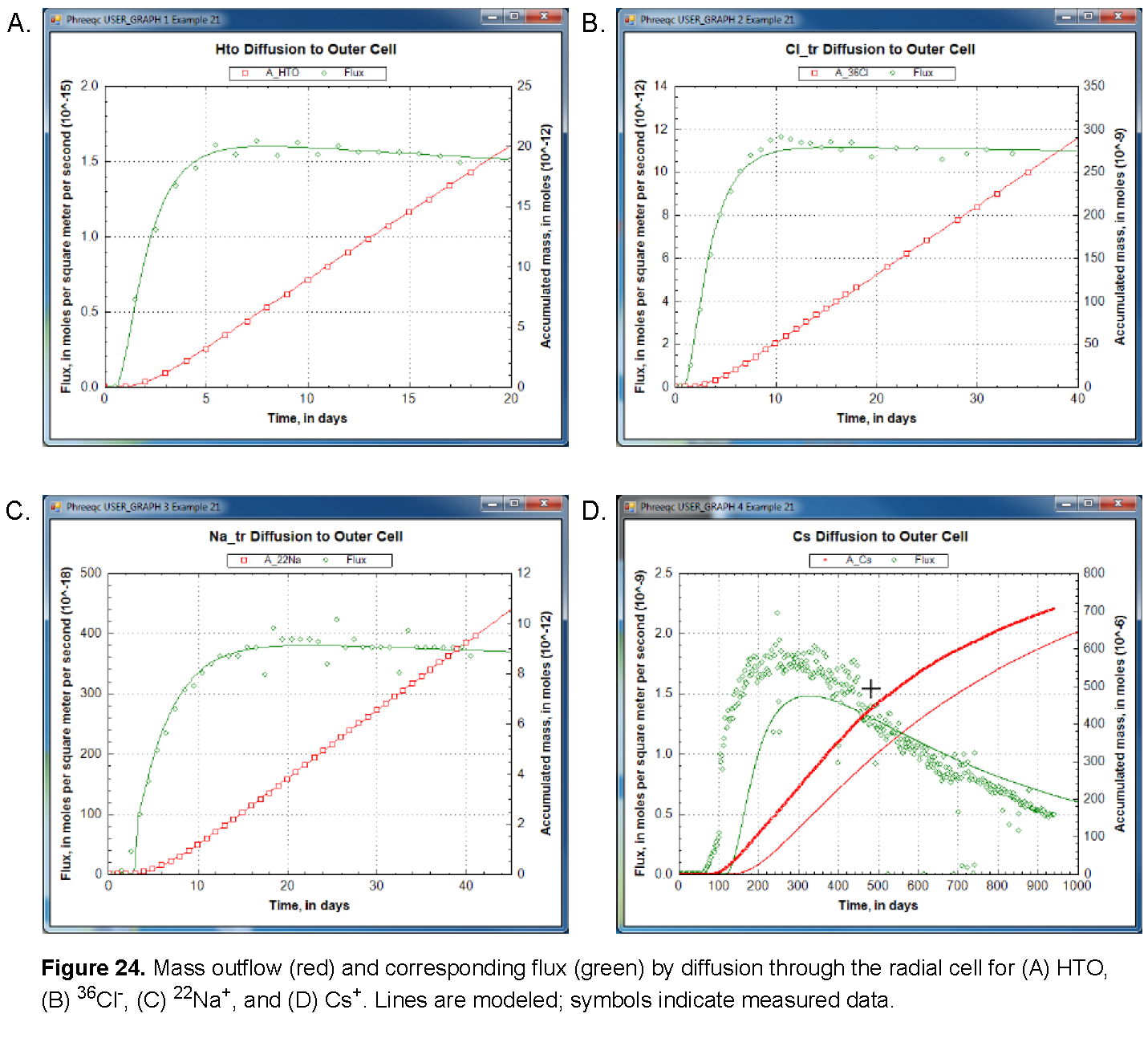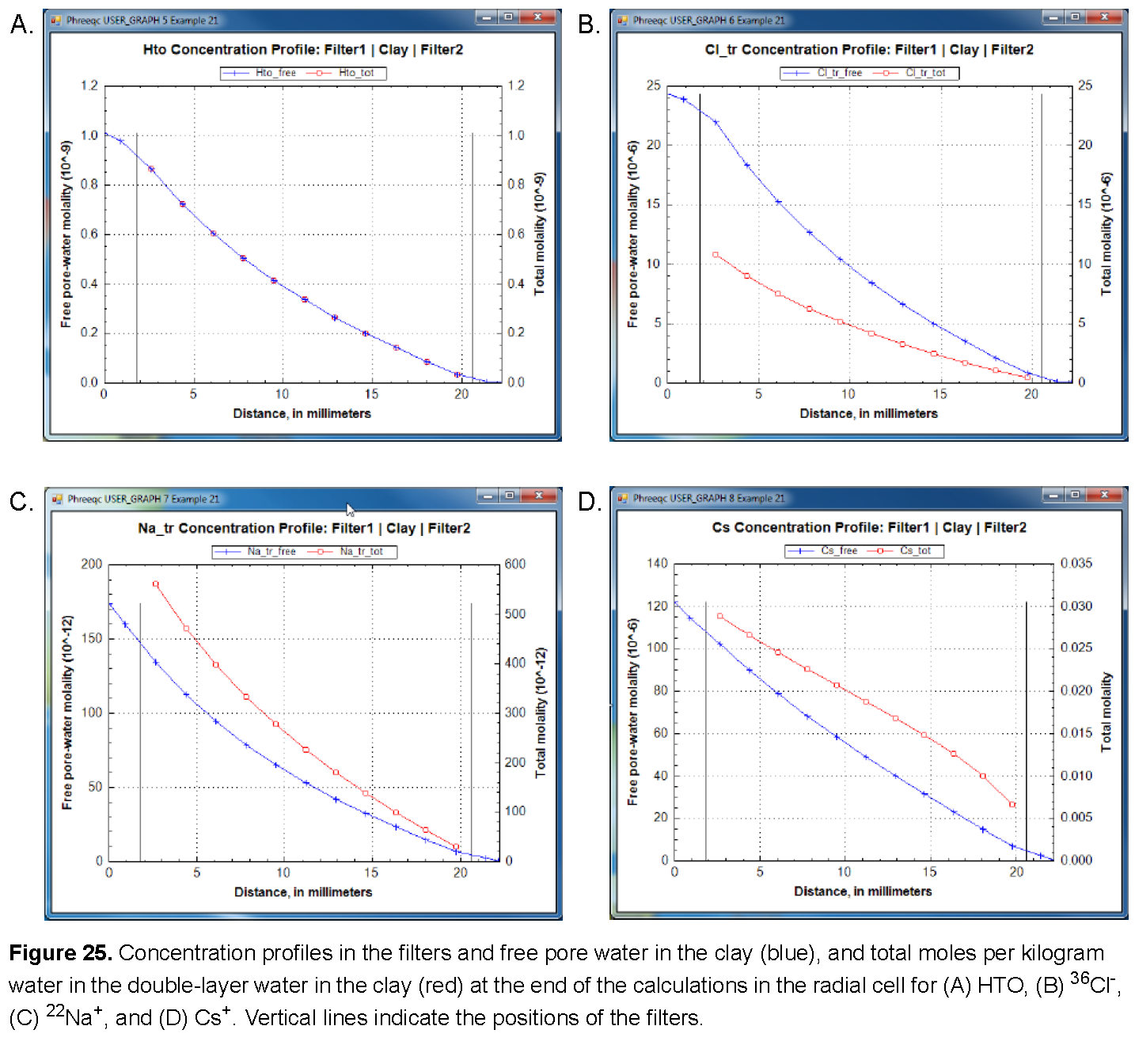The model simulates the experimental results very well (fig. 24), except for Cs+. The calculated arrival time of Cs+ is almost 100 days later than observed and then the mass accumulates too slowly. This behavior of Cs+ has been found in many similar experiments. It has been modeled by increasing the diffusion coefficient and decreasing the sorption capacity for Cs+ relative to batch experiments; these adjustments can be easily incorporated in this example.

`The diffusion of Cs+ can be increased by setting interlayer diffusion to true in Line 430: `
`  430  interlayer_D\$ = 'true'  `

(It is of interest to see the different effects of interlayer diffusion on 22Na+ and on Cs+.)

The results, shown in figure 26, illustrate that the arrival time of Cs+ can be matched by increasing diffusion, but that the mass accumulates too quickly. Another option for reducing the delay of the tracer arrival is to decrease the sorption of Cs+, either by decreasing the moles of surface and exchange sites or by decreasing the complexation constants, or both. By adjusting both the sorption and the diffusion coefficient, it may be possible to simulate the experimental data for Cs+. However, it remains difficult to explain why the sorption capacity is different between batch and diffusion experiments for Cs+, but not for the other cations.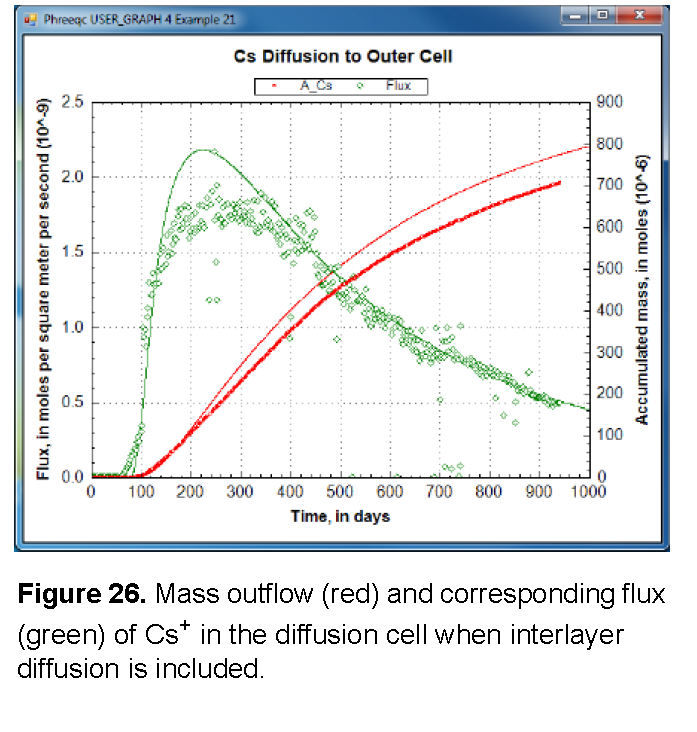Alternatively, and in line with the heterogeneous distribution of Cs+ in the clay after the experiment, the relatively fast arrival time can be modeled with a dual-porosity structure in which the pore space is subdivided into continuous and stagnant pores that can exchange by diffusion. The continuous pores allow Cs+ to move more rapidly through the clay than is calculated for a homogeneous medium, depending on the proportion, the flow velocity, and the diffusional exchange with the stagnant pores. With equation 49, and some effort, the dual-porosity structure can be introduced in the Basic program. Otherwise, the C program that is given as supplementary information in Appelo and others (2010) can be used. Similarly to the Basic program, the C program writes a complete PHREEQC input file for diffusion of Cs+, but in a dual porosity clay; in addition, the program permits distributing the surface and exchange sites differently between the stagnant and continuous pores.

| Next || Previous || Top |